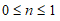# NMath User's Guide

38.5 Ranks, Percentiles, Deciles, and Quartiles (.NET, C#, CSharp, VB, Visual Basic, F#)

The static Ranks() method on class StatsFunctions returns the rank of each element in a data set an as array of integers. For example:

Code Example – C#

```int[] ranks  = StatsFunctions.Ranks( data );
```

Code Example – VB

```Dim Ranks() As Integer = StatsFunctions.Ranks(MyData)
```

By default, the ranks are calculated using ascending order. Alternatively, you can specify a sort order using a value from the enumeration. Thus:

Code Example – C#

```int[] ranks  =
```
```  StatsFunctions.Ranks( data, SortingType.Descending );
```

Code Example – VB

```Dim Ranks As Integer() = StatsFunctions.Ranks(MyData,
```
```  SortingType.Descending)
```

NOTE—StatsSettings.Sorting specifies the default SortingType.

The Rank() method returns where a given value would rank within a data set, if it were part of the data set. Again, the sorting order can be specified using a value from the enumeration. For instance:

Code Example – C#

```double x = 5.342;
```
```int rank = StatsFunctions.Rank( data, x, SortingType.Descending );
```

Code Example – VB

```Dim X As Double = 5.342
```
```Dim Rank As Integer = StatsFunctions.Rank(MyData, X,
```
```  SortingType.Descending)
```

Percentile() calculates the value at the nth percentile of the elements in a data set, where. For example, to find the value at the 95th percentile:

Code Example – C#

```double x = StatsFunctions.Percentile( data, 0.95 );
```

Code Example – VB

```Dim X As Double = StatsFunctions.Percentile(MyData, 0.95)
```

PercentileRank() performs the inverse calculation, returning the percentile a given value would have if it were part of the data set:

Code Example – C#

```double x = 23.653;
```
```double percentile = StatsFunctions.Percentile( data, x );
```

Code Example – VB

```Dim X As Double = 23.653
```
```Dim Percentile As Double = StatsFunctions.Percentile(MyData, X)
```

The returned percentile value is between 0 and 1.

Similarly, Decile() calculates a given decile, specified as an integer between 0 and 10, of the elements in a data set. Quartile() calculates a given quartile, specified as an integer between 0 and 4. For example, this code finds the third quartile value:

Code Example – C#

```double x = StatsFunctions.Quartile( data, 3 );
```

Code Example – VB

```Dim X As Double = StatsFunctions.Quartile(MyData, 3)
```

Top

Top